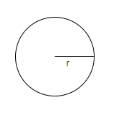QuestionAnswers

# Find the area of a quadrant of a circle whose circumference is 44cm.

Hint: Assume that the radius of the quadrant of the circle is ‘r’. Use the fact that the circumference of a circle with radius ‘r’ is $2\pi r$. Simplify the equation to calculate the radius of the circle. Calculate the area of a quadrant of the circle using the formula $\dfrac{\pi {{r}^{2}}}{4}$.

We have to calculate the area of a quadrant of the circle whose circumference is 44cm.
Let’s assume that the radius of the circle is ‘r’.
We know that the circumference of the circle with radius ‘r’ is $2\pi r$.Thus, we have $2\pi r=44$.
Simplifying the above expression, we have $r=\dfrac{44}{2\times \dfrac{22}{7}}$.
Rearranging the terms, we have $r=\dfrac{44\times 7}{2\times 22}=\dfrac{44\times 7}{44}=7cm$.
We will now calculate the area of the quadrant of a circle with radius equal to 7cm.
We know that the area of the quadrant of the circle with radius ‘r’ is $\dfrac{\pi {{r}^{2}}}{4}$.
Substituting $r=7cm$ in the above expression, the area of the quadrant of the circle $=\dfrac{\pi {{r}^{2}}}{4}=\dfrac{\pi }{4}{{\left( 7 \right)}^{2}}$.
Simplifying the above expression, the area of the quadrant of the circle is $=\dfrac{\dfrac{22}{7}}{4}\times 49=\dfrac{22\times 7}{4}=\dfrac{11\times 7}{2}=38.5c{{m}^{2}}$.
Hence, the area of the quadrant of the circle whose circumference is 44cm is $38.5c{{m}^{2}}$.

Note: We must keep in mind that we have data regarding the circumference of the circle and not the quadrant. If we will equate the circumference of the quadrant to 44cm, we will get an incorrect answer. We should also be careful about units while calculating the area of the quadrant of the circle. As the circumference is given in centimetres, the radius of the circle will be in centimetres as well. Thus the area of the quadrant of the circle will be in centimetres square.